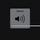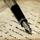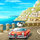## General Question# How do I solve this?

Asked by UnhappyPerson (20) 1 month ago

I need help with my math. This problem is really hard (for me). So here it is.

I am a number less than 3000. When you divide me by 32, my remainder is 30. When you divide me by 58, my remainder is 44. What number am I?

Observing members: 0Composing members: 0I don’t see how you can be asked to solve this unless you have studied modular arithmetic. Here is one simplification you should be able to understand. Let’s say that z is the number we are looking for. z = 58x + 32y. The advantage of expressing z like this is that it breaks the problem down into two smaller problems. Since 32y is divisible by 32, the remainder of z when divided by 32 is the same as the remainder of 58x when divided by 32. Similarly, the remainder of z when divided by 58 is the remainder of 32y when divided by 58.

Therefore we want to find x such that 58x has a remainder of 30 when divided by 32 and
we want to find y such that 32y has a remainder of 44 when divided by 58.

I found a trick for finding x. Unfortunately, I don’f know of an easy way to find y.

Part 1. Find x such that 58x has a remainder of 30 when divided by 32. 58x = 32x + 26x, so we want to find x such that 26x has a remainder of 30. Here is where the modular arithmetic comes in. 26 = -6 mod 32. Since (-5)x(-6)=30, x = -5 = 27 mod 32. If you do the arithmetic, you will see that in fact 58×27 has a remainder of 30 when dividing by 32.

Part 2. Find y such that 32y has a remainder of 44 when divided by 58. I know a way of finding y, but it is a bit complicated.

LostInParadise (27114)“Great Answer” (2) Flag as…Inspired_2write (11086)“Great Answer” (1) Flag as…Note that 928 is the least common multiple of 58 and 32. Since it is divisible by both 58 and 32, you can add or subtract it from any solution to get another number with the same remainders when divided by 32 and 58.

In what I did above, we can find y=5, so one solution is 5×32 + 27*58 = 1726. We can get a smaller solution by subtracting 928 from 1726 to get the smallest positive solution of 798, and so all solutions are of the form 798 + 928k.

LostInParadise (27114)“Great Answer” (1) Flag as…I was trying to figure out why I did not initially get the minimum solution. It turns out that 27 was not the smallest number x such that 58x has a remainder of 30 when divided by 32. 11 also works. The slick trick I used was not optimum, which can happen when you are not dividing by a prime. With x=11 and y=5, we get 58x + 32y = 798. I believe that the approach I used will always give the minimum solution, and as I showed above, adding the least common multiple gives the other solutions.

There is a generalization of what I did, referred to as the Chinese remainder theorem (which should give some idea of how long it has been around), that can be used to find solutions satisfying multiple equations of the form x has remainder ri when divided by mi. The math is not that difficult, but rather than try to explain it here, I recommend taking a quick glance at the relevant material in a book on number theory.

LostInParadise (27114)“Great Answer” (1) Flag as…Gee, you people are so clever! I will just crawl back into my shell.

ZEPHYRA (21437)“Great Answer” (0) Flag as…or